# Advanced Tutorial Lesson 4: Designing Low & High Frequency Oscillator Circuits

 Tutorial Project: Designing a Wien Bridge Oscillator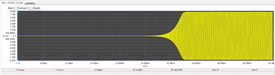Objective: In this project, you will design and realize a Wien bridge oscillator and analyze its operation. Concepts/Features: Operational Amplifier Wien Bridge Oscillation Frequency Barkhausen Criterion Positive Feedback Bipolar Junction Transistor Parallel LC Resonant Circuit Minimum Version Required: All versions

## What You Will Learn

In this tutorial you will first build a Wien Bridge Oscillator using an LM741 Op-Amp and will analyze its oscillatory behavior. Next, you will build and test a high frequency Colpitts oscillator using a parallel resonant LC circuit. The primary objective of this tutorial lesson is to underline the challenges of analyzing oscillator circuits.

## Designing a Wien Bridge Oscillator

The following is a list of parts needed for this part of the tutorial lesson:

Part Name Part Type Part Value
VCC DC Voltage Source 15V
VEE DC Voltage Source -15V
R1 - R3 Resistor 10k
R4 Resistor 20k
C1 - C2 Capacitor 1n
X1 LM741 Op-Amp Defaults

The Wien Bridge Oscillator comprises an Op-Amp, four resistors and two capacitors. The oscillator can also be viewed as a positive gain amplifier combined with a bandpass filter that provides positive feedback. At the resonant frequency, the reactance of the series R2–C2 arm will be an exact multiple of the shunt R1–C1 arm. If the two R3 and R4 arms are adjusted to the same ratio, then the bridge is balanced. These conditions can be written as:

$\omega^2 = \frac {1}{R_1 R_2 C_1 C_2}$

and

$\frac {C_1}{C_2} = \frac {R_4}{R_3} - \frac {R_2}{R_1}$

This can be further simplified by setting R1 = R2 = R and C1 = C2 = C. In that case, setting R4 = 2 R3 will satisfy the above conditions and will lead to the following simple equation for the oscillation frequency:

$f_o = \frac {1}{2 \pi RC}$

For this project, you will initially choose R1 = R2 = 10k, R3 = 10k, R3 = 2 R3 = 20k, and C1 = C3 = 100pF. Place and connect all the parts as shown in the above figure. Place a voltage probe marker at the output of the Op-Amp. The oscillation frequency of this circuit must be fo = 1 / (2π . 1e+4 . 1e-9) = 15.9kHz.

## Testing the Wien Bridge Oscillator

Run a Transient Test of this circuit with start and stop times set to 0 and 25ms and a Step Ceiling of 1μs. You will get a graph like the one shown below.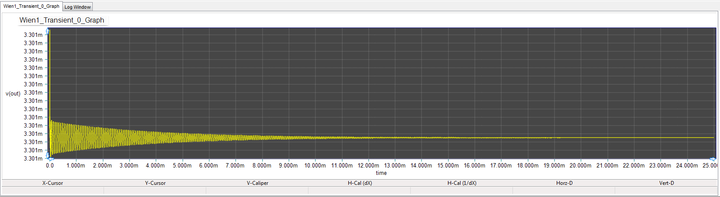The output voltage of the Wien Bridge Oscillator when R4 = 20k.

The circuit is certainly not oscillating in a sustainable way as the output voltage fluctuates around 3.3mV. Next, change the value of R4 to 21k and run a new Transient Test. This time you will get a fairly large oscillation between -5V and +5V as show below.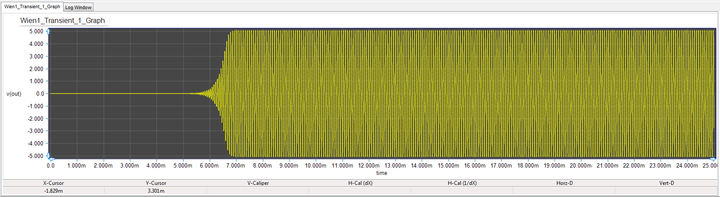The output voltage of the Wien Bridge Oscillator when R4 = 21k.

In order to calculate the oscillation frequency, first zoom in the graph window by clicking the "Zoom In" button of the Graph Toolbar. Then use the "Delta Tool" of the Graph Toolbar to measure the distance between two successive peaks of the sinusoidal signal. The period is about 78.5μs, which gives an oscillation frequency of 12.75kHz.Measuring the signal period on a close-up of the output voltage of the Wien Bridge Oscillator when R4 = 21k.

Next, reduce the value of R4 further to 20.25k and run a new Transient Test again, but this time with a stop time of 50ms. You will notice that the sustainable oscillations start at much later time around 30ms with a reduced peak-to-peak level. If you zoom in the graph and measure the signal period, you will note that it has reduced to 71.5μs, which gives an oscillation frequency of about 14kHz, fairly close to the design frequency.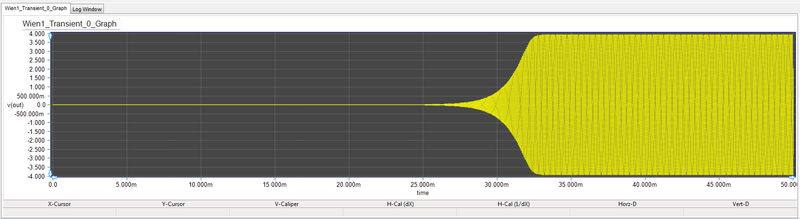The output voltage of the Wien Bridge Oscillator when R4 = 20.25k.

## Analyzing the Wien Bridge Oscillator and Explaining Its Behavior

The above behavior of the circuit can be explain using the feedback theory. The Wien Bridge Oscillator is indeed an non-inverting Op-Amp amplifier with positive feedback provided using two series and shunt RC impedances:

$Z_1(s) = \frac {R . \frac{1}{sC}}{R + \frac{1}{sC}}$

$Z_2(s) = R + \frac{1}{sC}$

The gain of the non-inverting amplifier is given by:

$A = 1 + \frac{R_4}{R_3}$

The feedback gain can be found from the voltage divider:

$\beta (s) = \frac{Z_1(s)}{Z_1(s) + Z_2(s)} = \frac {sRC} {s^2 R^2 C^2 + 3sRC + 1}$

The Barkhausen criterion for oscillation requires that T(s) = Aβ(s) ≥ 1. Substituting s = jω, one can write:

$T(j\omega) = A \beta(j\omega) = \frac {j\omega RC} {(1-\omega ^2 R^2 C^2)+ 3j\omega RC}$

A zero phase shift can be achieved only if

$1-\omega ^2 R^2 C^2 = 0$

which lead to the oscillation frequency ωo = 1/(RC). This further simplifies the Barkhausen criterion to

$T(j\omega) = \frac {A}{3} \ge 1$

which means that A = 1 + R4/R3 ≥ 3 or R4 ≥ 2R3. This shows that setting R4 = 2R3 provides the threshold of oscillation. For R4 > 2R3, positive feedback causes the oscillations to grow indefinitely.

## Designing a BJT Colpitts Oscillator

The following is a list of parts needed for this part of the tutorial lesson:

Part Name Part Type Part Value
VCC Voltage Source 12V
R1 Resistor 22k
R2 Resistor 10k
R3 Resistor 2k
R4 Resistor 1k
C1 Capacitor 100n
C2 - C3 Capacitor 1n
C4 Capacitor 27n
L1 Inductor 27u
Q1 NPN BJT 2N2222 beta = 150

To place the transistor part 2N222, open the "Parts Bin" by selecting the "Add Part" tab of the "Toolbox" on the left side panel. By default, the "Function" tab of the Part Bin is active. This means that parts are sorted based on their function. Open the "Active Components" menu and select the "Transistor..." item and from its submenu select the NPN... item as shown below. All the NPN BJT devices are listed in the Parts Bin. Scroll down the list and find and select “2N2222”. Either double-click the part's name or click the Place Part button at the bottom of the Parts Bin to place the device on your schematic.

The figure below shows a Colpitts oscillator:

The parallel resonant LC circuit consisting of L1 and capacitors C3 and C4 is connected between the base and the collector of transistor Q1, and partial feedback voltage is fed to its emitter through the voltage divider constituted by C3 and C4. The oscillating frequency of Colpitts oscillator can be calculated from:

$f_o = \frac {1}{2 \pi \sqrt{ L_1 \left( \frac{C_3 C_4}{C_3 + C_4} \right) } }$

Note that C1 and C2 are bypass and coupling capacitors, respectively. In this Colpitts oscillator circuit, the feedback factor β(s) is C3/(C3 + C4) ≅ C3/C4. The voltage gain A is given by:

$A = g_m R = \frac{I_C}{V_T} R_3 = \frac{kI_C}{qT} R_3$

Therefore, the Barkhausen criterion can be written as:

$A \beta(s) = \left( \frac{C_3}{C_3 + C_4} \right). \left( \frac{I_C}{V_T} \right). R_3 \ge 1$

## Building & Testing the Colpitts Oscillator

For this project, you will choose L1 = 27μH, C1 = 100nF, C2 = 1nF, C3 = 1nF, C4 = 15nF, R1 = 22k, R2 = 10k, R3 = 2k and R4 = 1k. Place and connect all the parts as shown in the above figure. Place a voltage probe marker at the output of the oscillator circuit at the collector of Q1. For the given inductance and capacitance value, the oscillation frequency of this Colpitts circuit is found to be fo = 1MHz.

Before running a Transient Test of your Colpitts oscillator, first run a DC Bias Test to find the DC operating point of the BJT. The results of the DC bias test are shown in the opposite figure. According to this table, the quiescent collector current IC is 2.985mA. The Barkhausen criterion in this case is thus satisfied:

$A \beta(s) = \left( \frac{1nF}{16nF} \right). \left( \frac{2.985\times 10^{-3}}{26\times 10^{-3}} \right). 2\times 10^3 = 14.351 \ge 1$

Now run a Transient Test of your oscillator circuit with start and stop times set at 0 and 100μs, respectively, and a Step Ceiling equal to 1ns. The output voltage graph is shown in the figure below. The output signal oscillates between 2V and 14V. A signal period of almost 1μs can be measured, which corresponds to an oscillation frequency of 1MHz.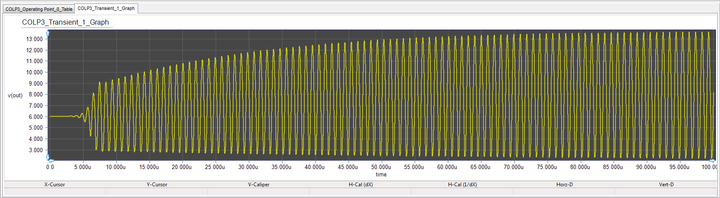The output voltage of the Colpitts Oscillator.

## Changing the Tuning of the Colpitts Oscillator

In this part, let's change the tuning of the parallel LC circuit to get an oscillation frequency of 4MHz. Due to the presence of the square root in the denominator of the formula for fo, you need to make the product of L1 and (C3 || C4) 16 times smaller. To do so, set C3 to 0.25nF and L1 to 6.75μH. Run a new transient test of your modified oscillator with the stop time set to 50μs. As you can see from the figure below, the output voltage this time oscillates between 3V and 11V with a period of about 260ns, which corresponds to an oscillation frequency of 3.85MHz.The output voltage of the modified Colpitts Oscillator with C3 = 0.25nF and L1 = 6.75μH.

Let's see what happen if you try to push the oscillation frequency even higher to 8MHz. For this purpose, let's reduce the value of C3 four times to 62.5pF. Run a new transient test of your oscillator with the stop time set to 50μs. The graph of the output voltage is shown in the figure blow. As you can see, the oscillation breaks down in this case.The output voltage of the modified Colpitts Oscillator with C3 = 62.5pF and L1 = 6.75μH.